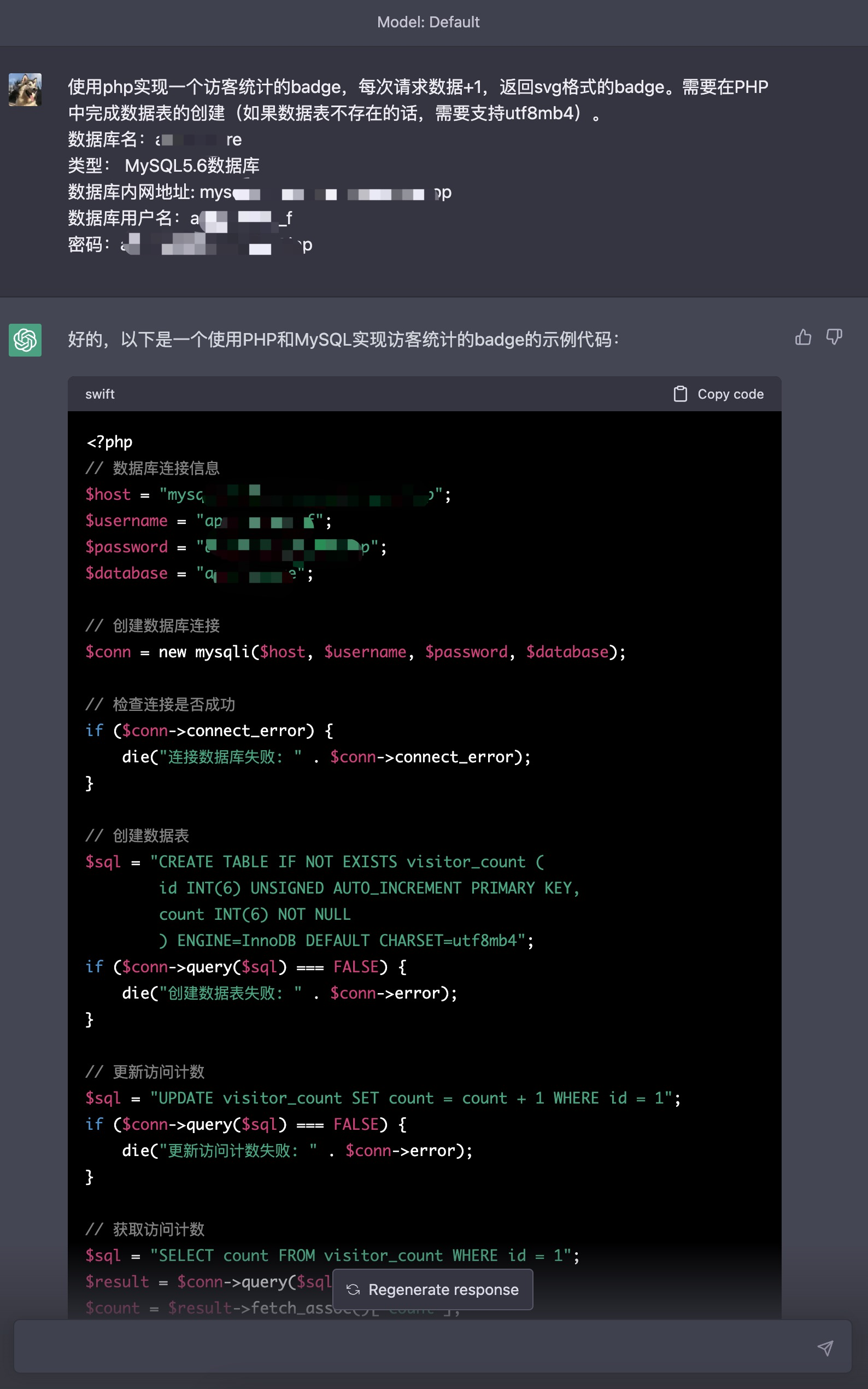•   更新：2023-03-01 19:41:11
•   首发：2023-02-27 22:30:00
•   源代码
•   200``````<?
// 数据库连接信息
\$host = "xxxxx";
\$database = "xxxxx";

// 创建数据库连接

// 检查连接是否成功
if (\$conn->connect_error) {
die("连接数据库失败: " . \$conn->connect_error);
}

// 创建数据库
\$sql = "CREATE DATABASE IF NOT EXISTS \$database CHARACTER SET utf8mb4 COLLATE utf8mb4_general_ci";
if (\$conn->query(\$sql) === FALSE) {
die("创建数据库失败: " . \$conn->error);
}

// 选择数据库
\$conn->select_db(\$database);

// 创建数据表
\$sql = "CREATE TABLE IF NOT EXISTS visitor_count (
id INT(6) UNSIGNED AUTO_INCREMENT PRIMARY KEY,
count INT(6) NOT NULL
) ENGINE=InnoDB DEFAULT CHARSET=utf8mb4";
if (\$conn->query(\$sql) === FALSE) {
die("创建数据表失败: " . \$conn->error);
}

// 关闭数据库连接
\$conn->close();
``````

``````// 更新访问计数
\$sql = "UPDATE visitor_count SET count = count + 1 WHERE id = 1";
if (\$conn->query(\$sql) === FALSE) {
die("更新访问计数失败: " . \$conn->error);
}

// 获取访问计数
\$sql = "SELECT count FROM visitor_count WHERE id = 1";
\$result = \$conn->query(\$sql);
\$count = \$result->fetch_assoc()["count"];

// 生成SVG徽章
echo <<<EOT
<svg xmlns="http://www.w3.org/2000/svg" width="130" height="20">
<stop offset="0" stop-color="#bbb" stop-opacity=".1"/>
<stop offset="1" stop-opacity=".1"/>
<rect width="130" height="20" rx="3" fill="#fff"/>
<path fill="#555" d="M0 0h74v20H0z"/>
<path fill="#4c1" d="M74 0h56v20H74z"/>
<path fill="url(#b)" d="M0 0h130v20H0z"/>
</g>
<g fill="#fff" text-anchor="middle" font-family="DejaVu Sans,Verdana,Geneva,sans-serif" font-size="11">
<text x="37" y="15" fill="#010101" fill-opacity=".3">Visitors</text>
<text x="37" y="14">Visitors</text>
<text x="105" y="15" fill="#010101" fill-opacity=".3">\$count</text>
<text x="105" y="14">\$count</text>
</g>
</svg>
EOT;

// 关闭数据库连接
\$conn->close();
``````

``````<img src="visitor_count.php" alt="Visitors" />
````````````![visitors](https://app.yizcore.xyz/badge.php?page_id=yi-ge)
``````

``````<?php
// 数据库连接信息
\$host = "";
\$database = "";

// 创建数据库连接

// 检查连接是否成功
if (\$conn->connect_error) {
die("连接数据库失败: " . \$conn->connect_error);
}

// 创建数据表
\$sql = "CREATE TABLE IF NOT EXISTS visitor_count (
id INT(6) UNSIGNED AUTO_INCREMENT PRIMARY KEY,
count INT(6) NOT NULL
) ENGINE=InnoDB DEFAULT CHARSET=utf8mb4";
if (\$conn->query(\$sql) === FALSE) {
die("创建数据表失败: " . \$conn->error);
}

// 更新访问计数
\$sql = "UPDATE visitor_count SET count = count + 1 WHERE id = 1";
if (\$conn->query(\$sql) === FALSE) {
die("更新访问计数失败: " . \$conn->error);
}

// 获取访问计数
\$sql = "SELECT count FROM visitor_count WHERE id = 1";
\$result = \$conn->query(\$sql);
\$count = \$result->fetch_assoc()["count"];

// 生成SVG徽章
echo <<<EOT
<svg xmlns="http://www.w3.org/2000/svg" width="130" height="20">
<stop offset="0" stop-color="#bbb" stop-opacity=".1"/>
<stop offset="1" stop-opacity=".1"/>
<rect width="130" height="20" rx="3" fill="#fff"/>
<path fill="#555" d="M0 0h74v20H0z"/>
<path fill="#4c1" d="M74 0h56v20H74z"/>
<path fill="url(#b)" d="M0 0h130v20H0z"/>
</g>
<g fill="#fff" text-anchor="middle" font-family="DejaVu Sans,Verdana,Geneva,sans-serif" font-size="11">
<text x="37" y="15" fill="#010101" fill-opacity=".3">Visitors</text>
<text x="37" y="14">Visitors</text>
<text x="105" y="15" fill="#010101" fill-opacity=".3">\$count</text>
<text x="105" y="14">\$count</text>
</g>
</svg>
EOT;

// 关闭数据库连接
\$conn->close();
?>
``````

上一篇 (【视频】ChatGPT完整实现定时请求小系统)